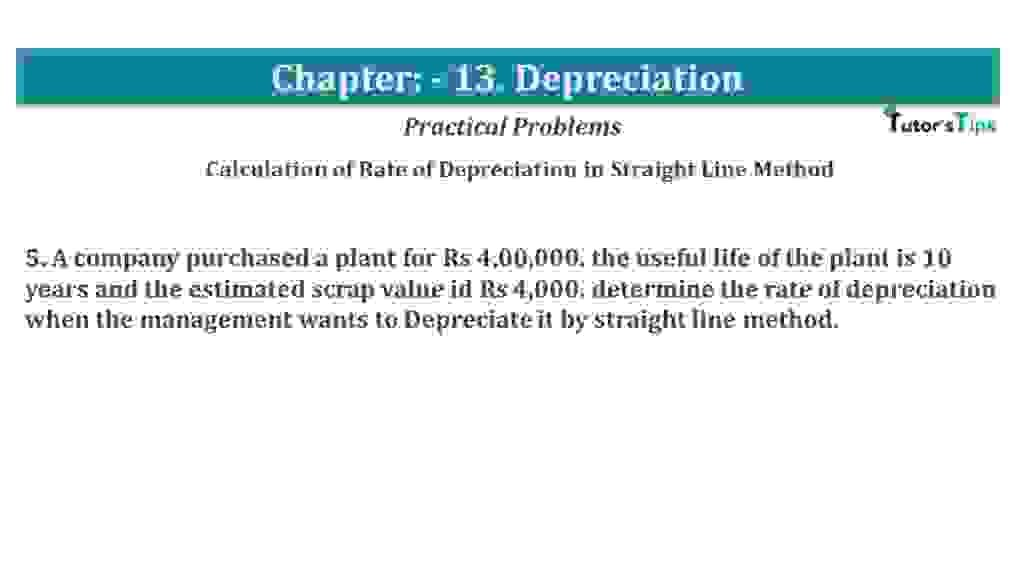# Question No 05 Chapter No 13 – USHA Publication 11 ClassQuestion No 05 Chapter No 13

Calculation of Rate of Depreciation in the Straight Line Method

5. A company purchased a plant for Rs 4,00,000. the useful life of the plant is 10 years and the estimated scrap value id Rs 4,000. determine the rate of depreciation when the management wants to Depreciate it by the straight-line method.

The solution of Question No 05 Chapter No 13:-

The method of calculating the Deprecation rate:

 Amount of Depreciation = Cost of assets- Estimated Realizable or Scrap value Number of years of expected useful life

Rate of Deprecation can be calculated with the help of following formula:

Cost of assets = Cost of Plant = 4,00,000
Number of years of expected useful life = 10 years
Estimated Retaliated or Scrap value = 40,000

 Amount of Depreciation = 4,00,000 – 40,000 = 36,000 10

Rate of Depreciation = 36,000 / 4,00,000 X 100 = 9%

Depreciation | Meaning | Methods | Examples

Thanks, Please Like and share with your friends

Comment if you have any question.

Also, Check out the solved question of all Chapters: –

Chapter No. 1 – Introduction

Chapter No. 2 – Theory Base of Accounting

Chapter No. 3 – Vouchers and transactions

Chapter No. 4 – Journal

Chapter No. 5 – Ledger

Chapter No. 6 – Cash Book

Chapter No. 7 – Other Subsidiary Books

Chapter No. 8 – Journal Proper

Chapter No. 9 – Trial Balance

Chapter No. 10 – Bank Reconciliation Statement

Chapter No. 11 – Depreciation

Chapter No. 12 – Provisions and Reserves

Chapter No. 13 – Bills of Exchange

Chapter No. 14 – Rectification of Errors

Chapter No. 15 – Financial Statements – (Without Adjustments)

Chapter No. 16 – Financial Statements – (With Adjustments)